# 7.5: How Do Chemical Reactions Occur? Reaction Rates

$$\newcommand{\vecs}{\overset { \rightharpoonup} {\mathbf{#1}} }$$ $$\newcommand{\vecd}{\overset{-\!-\!\rightharpoonup}{\vphantom{a}\smash {#1}}}$$$$\newcommand{\id}{\mathrm{id}}$$ $$\newcommand{\Span}{\mathrm{span}}$$ $$\newcommand{\kernel}{\mathrm{null}\,}$$ $$\newcommand{\range}{\mathrm{range}\,}$$ $$\newcommand{\RealPart}{\mathrm{Re}}$$ $$\newcommand{\ImaginaryPart}{\mathrm{Im}}$$ $$\newcommand{\Argument}{\mathrm{Arg}}$$ $$\newcommand{\norm}{\| #1 \|}$$ $$\newcommand{\inner}{\langle #1, #2 \rangle}$$ $$\newcommand{\Span}{\mathrm{span}}$$ $$\newcommand{\id}{\mathrm{id}}$$ $$\newcommand{\Span}{\mathrm{span}}$$ $$\newcommand{\kernel}{\mathrm{null}\,}$$ $$\newcommand{\range}{\mathrm{range}\,}$$ $$\newcommand{\RealPart}{\mathrm{Re}}$$ $$\newcommand{\ImaginaryPart}{\mathrm{Im}}$$ $$\newcommand{\Argument}{\mathrm{Arg}}$$ $$\newcommand{\norm}{\| #1 \|}$$ $$\newcommand{\inner}{\langle #1, #2 \rangle}$$ $$\newcommand{\Span}{\mathrm{span}}$$$$\newcommand{\AA}{\unicode[.8,0]{x212B}}$$

##### Learning Outcomes
• Define reaction rate.
• Explain the concept of activation energy.
• Label a diagram with reactants, products, enthalpy of forward and reverse reactions, activation energy of forward and reverse reactions, and activated complex.

Chemical kinetics is the study of the rates of chemical reactions. In this lesson, you will learn how to express the rate of a chemical reaction and about various factors that influence reaction rates.

## Expressing Reaction Rate

Chemical reactions vary widely in the speeds with which they occur. Some reactions occur very quickly. It a lighted match is brought into contact with lighter fluid or another flammable liquid, it erupts into flame instantly and burns fast. Other reactions occur very slowly. A container of milk in the refrigerator will be good to drink for weeks before it begins to turn sour. Millions of years were required for dead plants under Earth's surface to accumulate and eventually turn into fossil fuels such as coal and oil.

Chemists need to be concerned with the rates at which chemical reactions occur. Rate is another word for speed. If a sprinter takes 11.0 seconds $$\left( s \right)$$ to run a 100 meter (\left( m \right)\) dash, his rate or speed is given by the distance traveled divided by the time (see figure below).

$\text{speed} = \frac{\text{distance}}{\text{time}} = \frac{100 \: \text{m}}{11.0 \: \text{s}} = 9.09 \: \text{m/s}$

The sprinter's average running rate for the race is $$9.09 \: \text{m/s}$$. We say that it is his average rate because he did not run at that speed for the entire race. At the very beginning of the race, while coming from a standstill, his rate must be slower until he is able to get up to his top speed. His top speed must the be greater than $$9.09 \: \text{m/s}$$ so that taken over the entire race, the average ends up at $$9.09 \: \text{m/s}$$.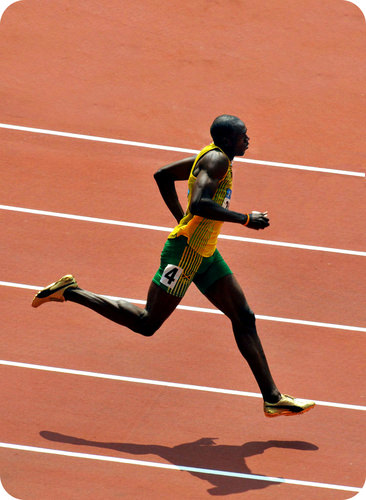Figure $$\PageIndex{1}$$: Usain Bolt set the world record for the 100 meter dash in 2009 with a time of 9.58 seconds. His average running rate over the course of this race was $$10.4 \: \text{m/s}$$, or $$23.4 \: \text{mph}$$.

Chemical reactions can't be measured in units of meters per second, as that would not make any sense. A reaction rate is the change in concentration of a reactant or product with time. Suppose that a simple reaction were to take place in which a 1.00 molar $$\left( \text{M} \right)$$ aqueous solution of substance $$\ce{A}$$ was converted to substance $$\ce{B}$$.

$\ce{A} \left( aq \right) \rightarrow \ce{B} \left( aq \right)$

Suppose that after 20.0 seconds, the concentration of $$\ce{A}$$ had dropped from $$1.00 \: \text{M}$$ to $$0.72 \: \text{M}$$ as it was being converted to substance $$\ce{B}$$. We can express the rate of this reaction as the change in concentration of $$\ce{A}$$ divided by the time.

$\text{rate} = -\frac{\Delta \left[ \ce{A} \right]}{\Delta t} = -\frac{\left[ \ce{A} \right]_\text{final} - \left[ \ce{A} \right]_\text{initial}}{\Delta t}$

A bracket around a symbol or formula means the concentration in molarity of that substance. The change in concentration of $$\ce{A}$$ is its final concentration minus its initial concentration. Because the concentration of $$\ce{A}$$ is decreasing over time, the negative sign is used. Thus, the rate for the reaction is positive, and the units are molarity per second or $$\text{M/s}$$.

$\text{rate} = -\frac{0.72 \: \text{M} - 1.00 \: \text{M}}{20.0 \: \text{s}} = -\frac{-0.28 \: \text{M}}{20.0 \: \text{s}} = 0.041 \: \text{M/s}$

Over the first 20.0 seconds of this reaction, the molarity of $$\ce{A}$$ decreases by an average rate of $$0.041 \: \text{M}$$ every second. In summary, the rate of a chemical reaction is measured by the change in concentration over time for a reactant or product. The unit of measurement for a reaction rate is molarity per second $$\left( \text{M/s} \right)$$.

## Collision Theory

The behavior of the reactant atoms, molecules, or ions is responsible for the rates of a given chemical reaction. Collision theory is a set of principles based around the idea that reactant particles form products when they collide with one another, but only when those collisions have enough kinetic energy and the correct orientation to cause a reaction. Particles that lack the necessary kinetic energy may collide, but the particles will simply bounce off one another unchanged. The figure below illustrates the difference. In the first collision, the particles bounce off one another, and no rearrangement of atoms has occurred. The second collision occurs with greater kinetic energy, and so the bond between the two red atoms breaks. One red atom bonds with the other molecule as one product, while the single red atom is the other produce. The first collision is called an ineffective collision, while the second collision is called an effective collision.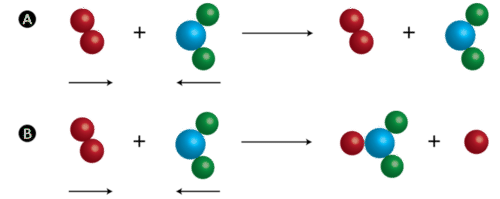Figure $$\PageIndex{2}$$: (A) An ineffective collision is one that does not result in product formation. (B) An effective collision is one in which chemical bonds are broken, and a product is formed.

Supplying reactant particles with energy causes the bonds between the atoms to vibrate with a greater frequency. This increase in vibrational energy makes a chemical bond more likely to break and a chemical reaction more likely to occur when those particles collide with other particles. Additionally, more energetic particles have more forceful collisions, which also increases the likelihood that a rearrangement of atoms will take place. The activation energy for a reaction is the minimum energy that colliding particles must have in order to undergo a reaction. Some reactions occur readily at room temperature because most of the reacting particles already have the requisite activation energy at that temperature. Other reactions only occur when heated because the particles do not have enough energy to react unless more is provided by an external source of heat.

### Potential Energy Diagrams

Then energy changes that occur during a chemical reaction can be shown in a diagram called a potential energy diagram, sometimes called a reaction progress curve. A potential energy diagram shows the change in the potential energy of a system as reactants are converted into products. The figure below shows basic potential energy diagrams for an endothermic (left) and an exothermic (right) reaction. Recall that the enthalpy change $$\left( \Delta H \right)$$ is positive for an endothermic reaction and negative for an exothermic reaction. This can be seen in the potential energy diagrams. The total potential energy of the system increases for the endothermic reaction as the system absorbs energy from the surroundings. The total potential energy of the system decreases for the exothermic reaction as the system releases energy to the surroundings.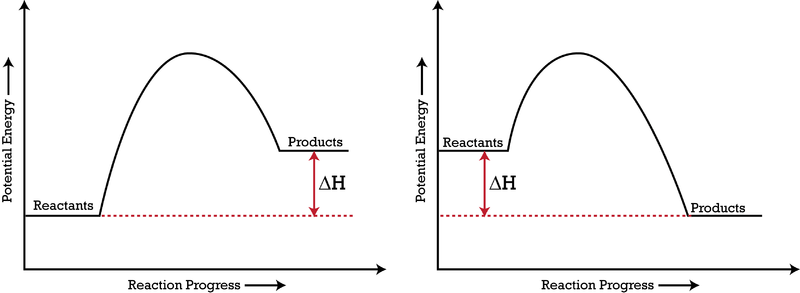Figure $$\PageIndex{3}$$: A potential energy diagram shows the total potential energy of a reacting system as the reaction proceeds. (Left) In an endothermic reaction, the energy of the products is greater than the energy of the reactants, and $$\Delta H$$ is positive. (Right) In an exothermic reaction, the energy of the products is lower than the energy of the reactants, and $$\Delta H$$ is negative.

The activation energy for a reaction is illustrated in the potential energy diagram by the height of the hill between the reactants and the products. For this reason, the activation energy of a reaction is sometimes referred to as the activation energy barrier. Reacting particles must have enough energy so that when they collide, they can overcome this barrier (see figure below).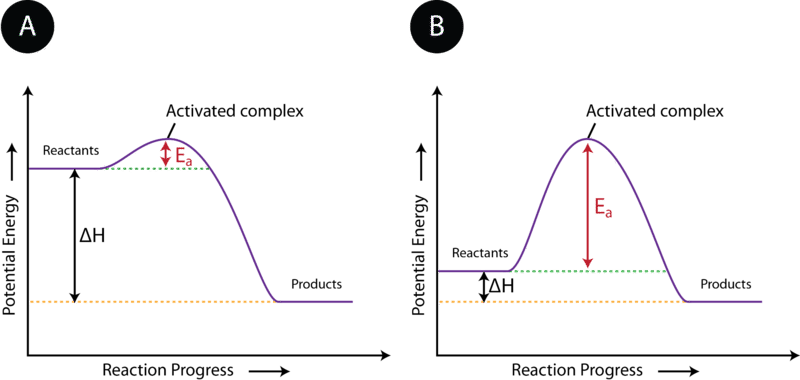Figure $$\PageIndex{4}$$: The activation energy $$\left( E_a \right)$$ of a reaction is the barrier that must be overcome in order for the reactants to become products. (A) The activation energy is low, meaning that the reaction is likely to be fast. (B) The activation energy is high, meaning that the reaction is likely to be slow.

As discussed earlier, reactant particles sometimes collide with one another and yet remain unchanged by the collision. Other times, the collision leads to the formation of products. The state of the particles that is in between the reactants and products is called the activated complex. An activated complex is an unstable arrangement of atoms that exists momentarily at the peak of the activation energy barrier. Because of its high energy, the activated complex exists only for an extremely short period of time (about $$10^{-13} \: \text{s}$$). The activated complex is equally likely to either reform the original reactants or go on to form the products. The figure below shows the formation of a possible activated complex between colliding hydrogen and oxygen molecules. Because of their unstable nature and brief existence, very little is known about the exact structures of most activated complexes.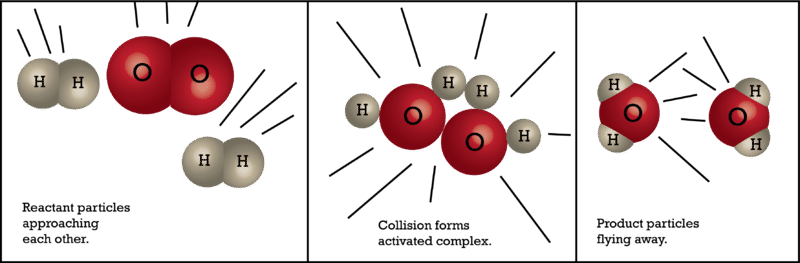Figure $$\PageIndex{5}$$: An activated complex is a short-lived state in which the colliding particles are at the peak of the potential energy curve.

7.5: How Do Chemical Reactions Occur? Reaction Rates is shared under a CC BY-NC-SA 3.0 license and was authored, remixed, and/or curated by LibreTexts.